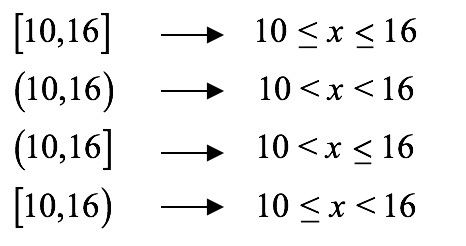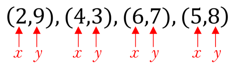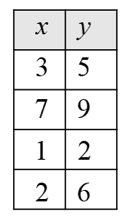MathCalculusConceptsA Guide to Set Builder Notation

# A Guide to Set Builder Notation

## Define a Set Notation

A set is a collection of elements. There are different ways in which elements listed in a set may be expressed. Set notations vary according to the characteristics of the set. Typically, a capital letter is used to represent a set. A lowercase letter may be used to represent the elements in the given set. For example:

X = {all natural numbers}

Y = {1, 2, 3, 4, 5…}

Z =

Set X, Set Y and Set Z refer to the same set of elements.

Set Y is a form that is referred to as roster notation. In this type of set notation, the elements are listed inside braces (curly brackets) separated by commas. The three dots following the number 5 are the ellipsis, used to indicate that the sequence continues.

Set Z is called the set builder notation. In set builder notation, we use instruction rather than any sentences or numbers. The instructions to writing a set in a set builder notation are:

• Pick any lowercase letter to denote the elements of the set.
• Place a vertical bar after the lowercase letter.
• Write the mathematical instructions on how the lowercase letter behaves under the given criteria.

In the above example, Set Z is read out as “set of all a, such that a is an element of natural numbers”.

In addition to natural numbers, there are other types of numbers which have their symbols as well. A few of them are listed below:

R: real numbers

N: natural numbers

Z: integers

Q: rational numbers

C: complex numbers

## Interval Notation

An Interval is a set of numbers lying between two given numbers. Interval notation refers to writing the start and end of the interval. We use two types of brackets; square [ ] and round ( ). Use a square bracket [ ] when the end values of the set are to be included. Square brackets are also referred to as closed brackets. The round brackets ( ) are used when the end values are not to be included and are also called open brackets. Consider the examples given below with different combinations of square and round brackets.

[10, 16]: Closed interval, values between 10 and 16, as well as end values - that is, 10 and 16 are included.

(10, 16): Open interval, values between 10 and 16 are included, and end values – that is, 10 and 16 are NOT included.

(10, 16]: Open interval on the left, closed interval on the right. Values between 10 and 16 are included. End value 16 is included, but 10 is NOT included.

[10, 16): Closed interval on the left, open interval on the right. Values between 10 and 16 are included. End value 10 is included but 16 is NOT included

Accordingly, we express the interval notations for the examples above on number lines as:

## Set Builder Notation and Interval Notations

Set builder notation can also be expressed in the form of inequalities as shown below.We know that $\infty$ a never-ending value. It cannot be contained. Therefore, if a given set has $\infty$ as the end values, then use open brackets for $\infty$.

For example

• Set of all-natural numbers in roster form is written as A = {1, 2, 3, 4, 5...}. The same set in set builder notation will be expressed as A = [1,∞)
• Set of all negative integers in set builder notation is B = (-∞,-1]
• Set of values of y in the expression y = x+1 in set builder notation is (-∞,∞)

## Set Notation

• If two sets A and B are combined, it is called union of the sets. It is denoted by symbol ꓴ. This symbol is used when the sets are expressed in interval notation.
• In the example below, we have two sets. First set A denoted by the blue line has elements between 4 and 6 while the second set in red has elements between 8 and 12. The element 7 is missing in both the sets. The combined set on a common number line can be seen on the number line between 4 and 12.

• If there are two sets with a few common elements in them, we use a symbol ∩ called intersection. In the example below, we have two sets. First set A denoted by the blue line has elements between -1 to 4 while the second set in red has elements between 1 and 6. The elements 1, 2, 3, 4 are common for both the sets. The overlap can be seen on the number line between 1 and 4.
• If there are two sets wherein one set is inside the other set, then the second set is called a subset of the first set. For example

A = {1, 2, 3, 4, 5} B = {3, 4, 5}

Then we say that B is a subset of A. It is denoted as B⊆A

## Set Notation Domain and Range

Domain and range establish the relationship between x and y values of ordered pairs. Domain is the set of values that x can take, and range is the set of values that y can take.

Consider the set of ordered pairs shown below.The domain for above set of ordered pairs will be: {2, 4, 5, 6}

The range for above set of ordered pairs is given as: {3, 7, 8, 9}

Note that domain and range are always written in an increasing order.

The domain and range for the mapping diagram will be

Domain: {1, 3, 6, 7}
Range: {2, 4, 8, 9}

The domain and range for the tabular values is given as

Domain: {1,2,3,7}
Range: {2, 5, 6, 9}The domain and range for the graphical values is given as

Domain: {-3, -1, 2, 4}
Range: {-1, 2, 3, 4}

## Set Builder Notation Summary

Advantages of Using Set Builder Notation:

A set builder notation is helpful when the number of elements in each set are large and follow a pattern.

Let us consider an example and write a set of positive even numbers that is less than 11 using roster, as well as, set builder notation.

Roster Notation:

Set Builder Notation:

### Want more help with your calculus homework?

We've got you covered with step-by-step solutions to millions of textbook problems, subject matter experts on standby 24/7 when you're stumped, and more.
Check out a sample calculus Q&A solution here!

*Response times vary by subject and question complexity. Median response time is 34 minutes and may be longer for new subjects.

### Search. Solve. Succeed!

Study smarter access to millions of step-by step textbook solutions, our Q&A library, and AI powered Math Solver. Plus, you get 30 questions to ask an expert each month.

Tagged in
MathCalculus

### Fundamental of Calculus